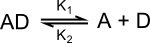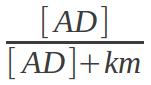# Enzyme kinetics

13 Sep 2011

As I mentioned in the previous section about reactions, in order for any reaction to occur in my simulation, an enzyme is required to catalyse it. This potentially reduces the possibilities for interesting chemistry to evolve, but simplifies computation.

Enzymes are added to Solution objects (including Cell objects) with the addProtein() function. For example, continuing from the code in the previous post:

``pool.addProtein(amount=2.0, sequence='MLMMMM')``

Here I've added two units of a protein with the amino acid sequence 'MLMMMM' to the pool. The 15 amino acids in my simulation are represented by the letters L to Z. The amino acid sequence 'MLMMMM' codes for a type of enzyme I call ADases because they catalyse the hydrolysis/synthesis of chemical AD to/from chemicals A and D. In a later post I'll explain exactly how amino acid sequences define protein functions.

Once we have set up a pool of water, we can run the simulation for multiple ticks like so:

``pool.update(ticks=1000)``

This graph shows how the concentrations change over 1000 ticks in a solution of 1% AD, 0.5% A and 0% D when 0.01% ADase is added.

The net reaction is that AD is hydrolysed until equilibrium is reached. Equilibrium is reached when the rate of AD hydrolysis occurs at the same rate of AD synthesis. In this case, equilibrium is reach when approximately 97% of the AD has been broken down.

### Rate constants

The relative rates of the forward and reverse reactions depends on the concentrations of the chemicals involved and two rate constants. These rate constants (k1 and k2) are defined by the relative stabilities of the chemicals on either side of the equation.The hydrolysis of AD into A and D has a rate constant (k1) of 0.6. If the reaction were to occur spontaneously, this would result in 60% of AD in the pool breaking down in each tick of the simulation. However, since this reaction requires an enzyme to catalyse it, the rate is multiplied by the amount of AD bound the enzyme. This equation shows how I defined the amount of AD bound to the enzyme at any given point in time.Here [AD] is the concentration of AD, i.e. the amount of AD divided by the volume of the solution. The km is a constant associated with each enzyme, which determines its affinity for its substrates. The amount of AD bound is actually slightly less in the simulation as AD competes for the enzyme binding site with A and D.

The reverse reaction is more complicated as it depends on two substrates. The equation is essentially the same, but is limited by whichever substrate is the least bound to the enzyme. This is a bit of a cheat, but simplifies things and stops reaction rates becoming vanishingly small when many substrates are involved.

### The effect of km

The km of an enzyme in my simulation, and in reality, determines how the concentration of a substrate affects the reaction rate. From the equation above, you can see that when the concentration of a substrate is equal to the km, half the enzyme will be bound to the substrate. A small km therefore means that an enzyme binds a substrate tightly and saturates at a relatively low concentration. A large km means that an enzyme binds the substrate less tightly and saturates at a higher concentration. Enzymes with a low km will catalyse reactions faster but will be less responsive to changes in substrate concentration.

This graph shows how substrate concentration affects the reaction rate of four ADase enzymes with different kms. As the substrate concentration increases, the reaction rate asymptotically reaches the maximum rate of 0.6. Only the initial reaction rate is measured so there is no reverse reaction or product inhibition.

### Comparison of reaction rates

As I mentioned above, rate constants depend on the relative stability of the chemicals involved in a reaction. AD is relatively unstable so equilibrium is reached when most of it (~97%) has been broken down. On the other hand, chemical FG is relatively stable, so breaks down slowly and reaches equilibrium at a higher concentration (only ~20% broken down). EH is somewhere in between.